As per given figure to complete the circular loop what should be the radius if initial height is 5?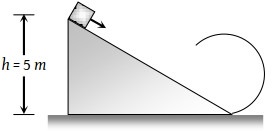(1) 4 m

(2) 3 m

(3) 2.5 m

(4) 2 m

Concept Questions :-

Work-Energy theorem
High Yielding Test Series + Question Bank - NEET 2020

Difficulty Level:

The kinetic energy k of a particle moving along a circle of radius R depends on the distance covered s as k = as2 where a is a constant. The force acting on the particle is

(1) $2a\frac{{s}^{2}}{R}$

(2) $2as{\left(1+\frac{{s}^{2}}{{R}^{2}}\right)}^{1/2}$

(3) 2 as

(4) $2a\frac{{R}^{2}}{s}$

High Yielding Test Series + Question Bank - NEET 2020

Difficulty Level:

A stone of mass 1 kg tied to a light inextensible string of length $L=\frac{10}{3}m$ is whirling in a circular path of radius L in a vertical plane. If the ratio of the maximum tension in the string to the minimum tension in the string is 4 and if g is taken to be 10 m/sec2, the speed of the stone at the highest point of the circle is

(1) 20 m/sec

(2) $10\sqrt{3}m/\mathrm{sec}$

(3) $5\sqrt{2}\text{\hspace{0.17em}}m/\mathrm{sec}$

(4) 10 m/sec

Concept Questions :-

Work-Energy theorem
High Yielding Test Series + Question Bank - NEET 2020

Difficulty Level:

A small block is shot into each of the four tracks as shown below. Each of the tracks rises to the same height. The speed with which the block enters the track is the same in all cases. At the highest point of the track, the normal reaction is maximum in:

 (1)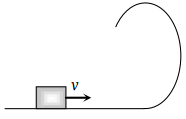(2)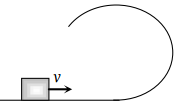(3)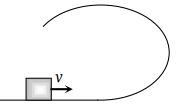(4) Same in all cases

Concept Questions :-

Work-Energy theorem
High Yielding Test Series + Question Bank - NEET 2020

Difficulty Level:

A stone tied to a string of length L is whirled in a vertical circle with the other end of the string at the centre. At a certain instant of time, the stone is at its lowest position and has a speed u. The magnitude of the change in its velocity as it reaches a position where the string is horizontal is:

(1) $\sqrt{{u}^{2}-2gL}$

(2) $\sqrt{2gL}$

(3) $\sqrt{{u}^{2}-gl}$

(4) $\sqrt{2\left({u}^{2}-gL\right)}$

Concept Questions :-

Work-Energy theorem
High Yielding Test Series + Question Bank - NEET 2020

Difficulty Level:

The driver of a car travelling at velocity v suddenly see a broad wall in front of him at a distance d. He should

(1) Brake sharply

(2) Turn sharply

(3) (1) and (2) both

(4) None of the above

Concept Questions :-

Concept of work
High Yielding Test Series + Question Bank - NEET 2020

Difficulty Level:

Which of the following graph depicts spring constant k versus length l of the spring correctly

 (1)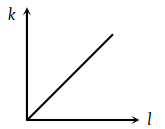(2)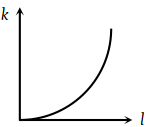(3)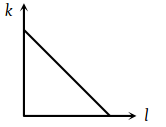(4)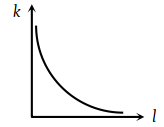Concept Questions :-

Elastic potential energy
High Yielding Test Series + Question Bank - NEET 2020

Difficulty Level:

Work done by a frictional force is

(1) Negative

(2) Positive

(3) Zero

(4) All of the above

Concept Questions :-

Concept of work
High Yielding Test Series + Question Bank - NEET 2020

Difficulty Level:

A block of mass 50 kg slides over a horizontal distance of 1 m. If the coefficient of friction between their surfaces is 0.2, then work done against friction is

(1) 98 J

(2) 72J

(3) 56 J

(4) 34 J

Concept Questions :-

Concept of work
High Yielding Test Series + Question Bank - NEET 2020

Difficulty Level:

A body of mass m is moving in a circle of radius r with a constant speed v. The force on the body is $\frac{m{v}^{2}}{r}$ and is directed towards the centre. What is the work done by this force in moving the body over half the circumference of the circle

(1) $\frac{m{v}^{2}}{\pi {r}^{2}}$

(2) Zero

(3) $\frac{m{v}^{2}}{{r}^{2}}$

(4) $\frac{\pi {r}^{2}}{m{v}^{2}}$

Concept Questions :-

Concept of work